﻿ (2 + 1)-维K-P方程精确解的研究 Study on Exact Solutions of (2 + 1)-Dimensional K-P Equation

Vol.05 No.03(2016), Article ID:18445,5 pages
10.12677/AAM.2016.53056

Study on Exact Solutions of (2 + 1)-Dimensional K-P Equation

Huifang Wei

School of Mathematics and Statistics, Yunnan University of Finance and Economics, Kunming Yunnan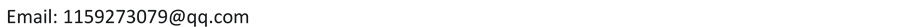Received: Aug. 17th, 2016; accepted: Aug. 27th, 2016; published: Aug. 30th, 2016ABSTRACT

In this paper, we mainly use the dynamical systems method to solve the (2 + 1) dimensional KP equation. By introducing the traveling wave transformation, the original (2 + 1) dimensional KP equation is transformed into ordinary differential equations. After that, we analyze the singular points, the corresponding bifurcation and phase diagram of the system. Finally, the solution of the original equation is obtained by using the Maple software, including the sharp wave solutions and periodic wave solutions.

Keywords:Dynamical Systems, Sharp Wave Solution, K-P Equation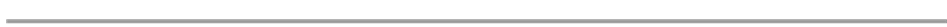(2 + 1)-维K-P方程精确解的研究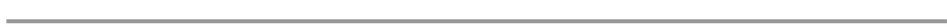1. 引言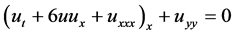(1)

2. 偏微化常微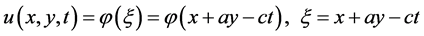(2)(3)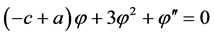(4)

(4)式等价于如下的二维系统：(5)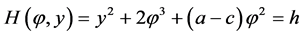(6)

3. 分析系统(5)的分支和相图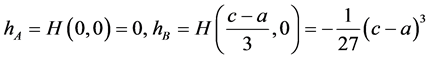，对于奇点的类型，我们还需要通过系统(5)对应的雅克(7)

1) 当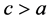时，A为鞍点，B为中心，见图1

2) 当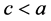时，A为中心，B为鞍点，见图2

3) 当时，仅为一个奇点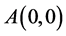，见图3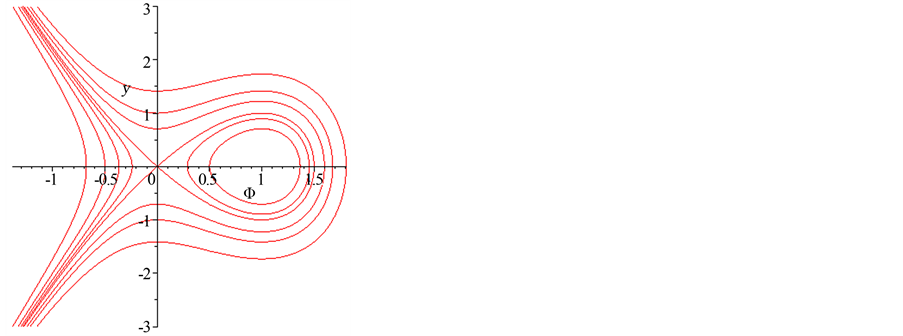Figure 1. c > a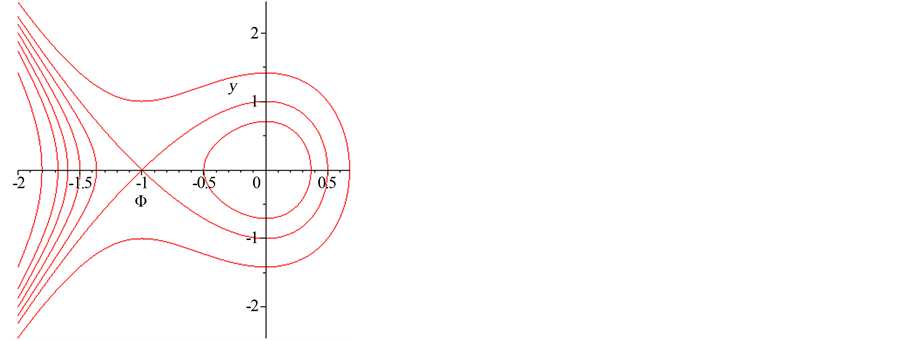Figure 2. c < a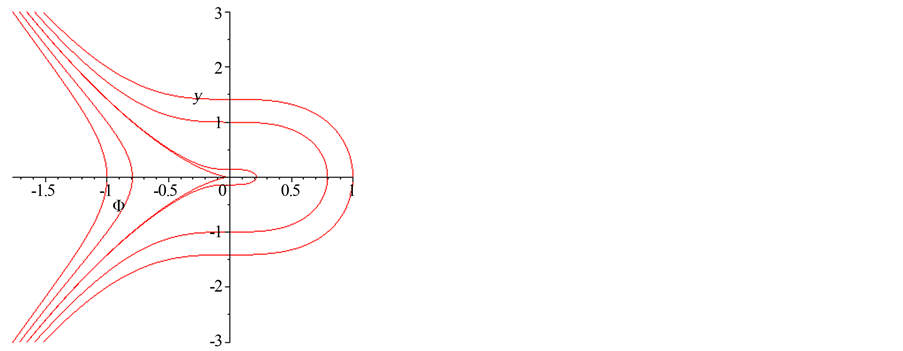Figure 3. c = a

4. 方程的求解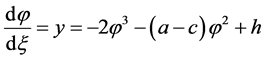(8)

1) 当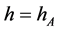时，带入(8)后，借助Maple两边积分一次，可得孤立波解(9)

2) 当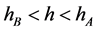时，带入(8)后，借助Maple两边积分一次，可得周期波解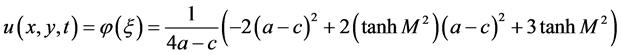(10)

3) 当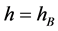时，带入(8)后，借助Maple两边积分一次，可得孤立波解(11)

4) 当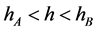时，带入(8)后，借助Maple两边积分一次，可得周期波解为(12)

5. 结论

Study on Exact Solutions of (2 + 1)-Dimensional K-P Equation[J]. 应用数学进展, 2016, 05(03): 450-454. http://dx.doi.org/10.12677/AAM.2016.53056

1. 1. 唐晓芬. 非线性偏微分方程的几类求解方法[D]: [硕士学位论文]. 镇江: 江苏大学, 2009.

2. 2. Bilige, S. and Chaolu, T. (2011) A Generalized (G’/G)-Expansion Method and Its Applications. Journal of Mongolia University Natural Science Edition, 1, 15-37.

3. 3. 徐振民. 推广的Tanh-函数法及其应用[J]. 广西民族大学学报(自然科学版), 2009, 3(15): 54-56.

4. 4. 刘涛立. F-展开法研究[D]: [硕士学位论文]. 兰州: 兰州大学, 2004.

5. 5. 闵迪. 非线性发展方程的求解与达布变换[D]: [硕士学位论文]. 大连: 辽宁师范大学, 2010.

6. 6. 杨攀攀. 齐次平衡法和非线性偏微分方程的孤立波解[D]: [硕士学位论文]. 南京: 南京理工大学, 2008.

7. 7. Li, J.B. (2013) Singular Nonlinear Traveling Wave Equations: Bifurcations and Exact Solutions. Science Press, Beijing, 1-70.

8. 8. 杨翠平. (3+1)维KP方程的N-孤子解[J]. 湖北广播电视大学学报, 2010, 12(30): 160-162.

9. 9. 傅海明, 戴正德. (3+1)维K-P方程的周期波解[J]. 山西大学学报(自然科学报), 2010, 33(1): 4-7.